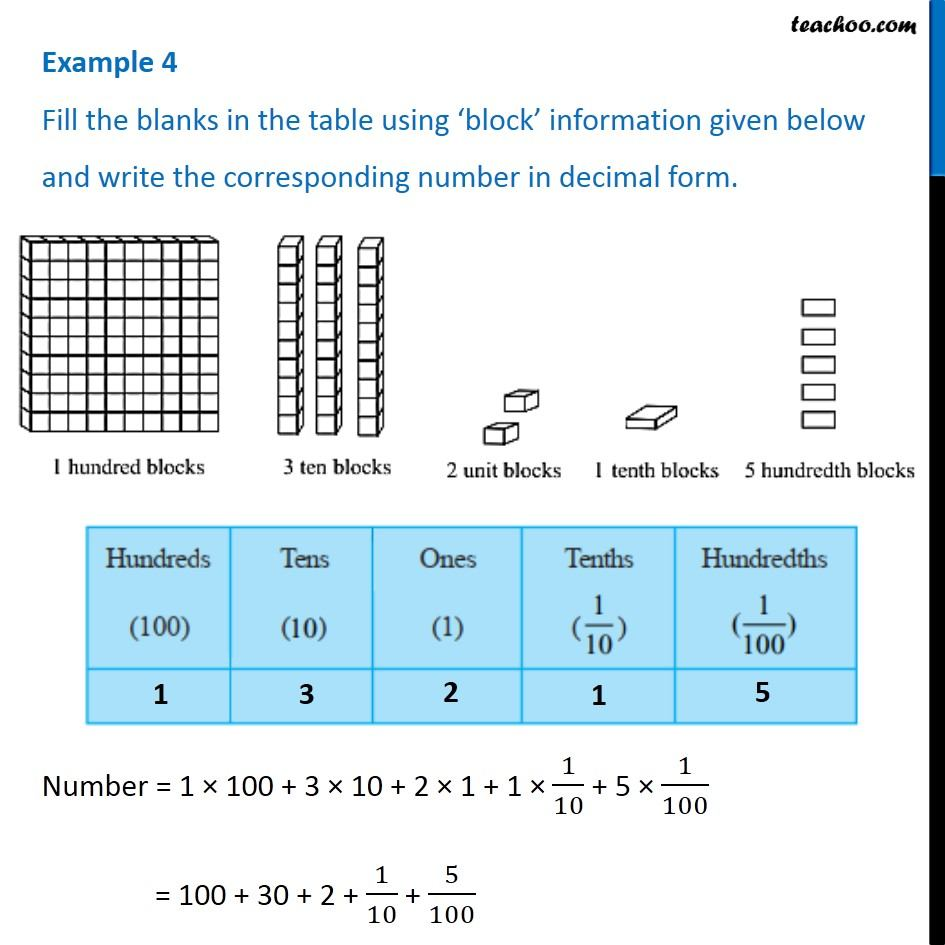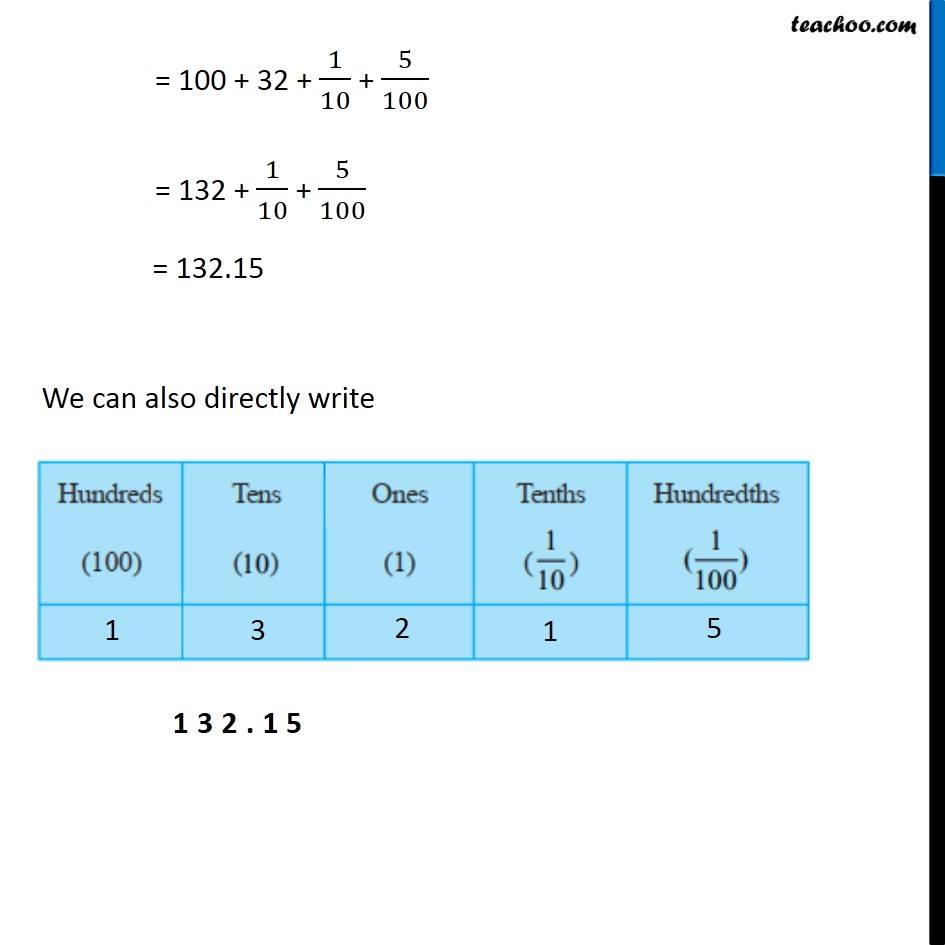Examples

Chapter 8 Class 6 Decimals
Serial order wiseLearn in your speed, with individual attention - Teachoo Maths 1-on-1 Class

### Transcript

Question 4 Fill the blanks in the table using ‘block’ information given below and write the corresponding number in decimal form. Number = 1 × 100 + 3 × 10 + 2 × 1 + 1 × 1/10 + 5 × 1/100 = 100 + 30 + 2 + 1/10 + 5/100 = 100 + 32 + 1/10 + 5/100 = 132 + 1/10 + 5/100 = 132.15 We can also directly write